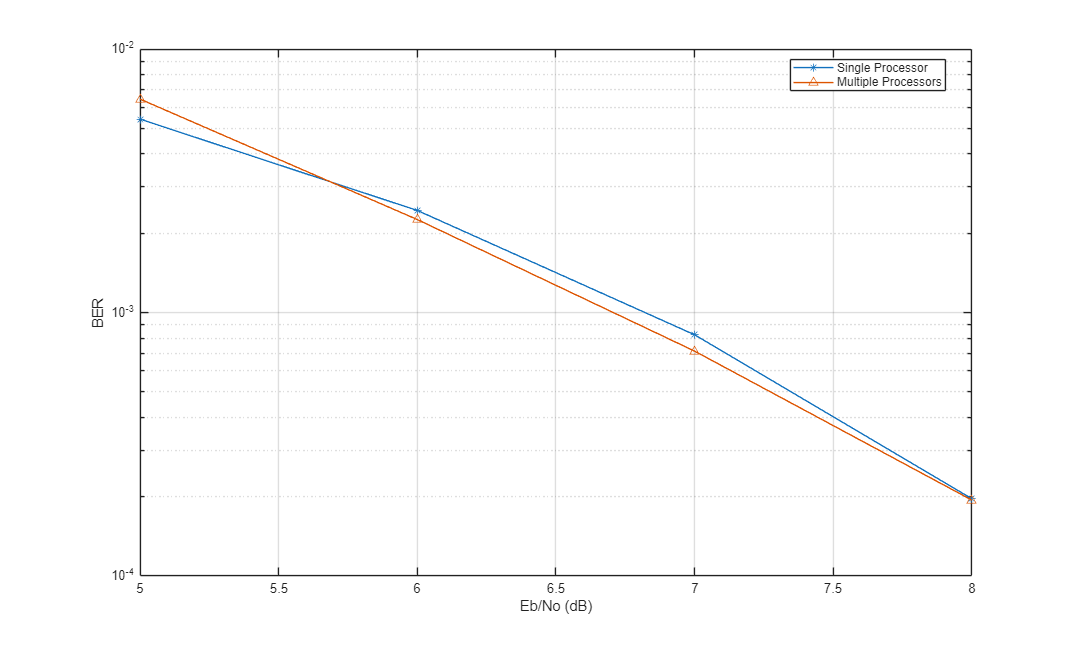Documentation

### This is machine translation

Mouseover text to see original. Click the button below to return to the English version of the page.

Note: This page has been translated by MathWorks. Click here to see
To view all translated materials including this page, select Country from the country navigator on the bottom of this page.

## Accelerating BER Simulations Using the Parallel Computing Toolbox

This example shows how to use the Parallel Computing Toolbox to accelerate a simple, QPSK bit error rate (BER) simulation. The system consists of a QPSK modulator, a QPSK demodulator, an AWGN channel, and a bit error rate counter. In this example, four parallel processors are used.

Set the simulation parameters.

```EbNoVec = 5:8; % Eb/No values in dB totalErrors = 200; % Number of bit errors needed for each Eb/No value totalBits = 1e7; % Total number of bits transmitted for each Eb/No value ```

Allocate memory to the arrays used to store the data generated by the function, `helper_qpsk_sim_with_awgn`.

```[numErrors, numBits] = deal(zeros(length(EbNoVec),1)); ```

Run the simulation and determine the execution time. Only one processor will be used to determine baseline performance. Accordingly, observe that the normal for-loop is employed.

```tic for idx = 1:length(EbNoVec) errorStats = helper_qpsk_sim_with_awgn(EbNoVec, idx, ... totalErrors, totalBits); numErrors(idx) = errorStats(idx,2); numBits(idx) = errorStats(idx,3); end simBaselineTime = toc; ```

Calculate the BER.

```ber1 = numErrors ./ numBits; ```

Rerun the simulation for the case in which the Parallel Computing Toolbox is available. Create a pool of workers.

```pool = gcp; ```

Determine the number of available workers from the `NumWorkers` property of `pool`. The simulation runs the range ofvalues over each worker rather than assigning a singlepoint to each worker as the former method provides the biggest performance improvement.

```numWorkers = pool.NumWorkers; ```

Determine the length of `EbNoVec` for use in the nested `parfor` loop. For proper variable classification, the range of a for-loop nested in a `parfor` must be defined by constant numbers or variables.

```lenEbNoVec = length(EbNoVec); ```

Allocate memory to the arrays used to store the data generated by the function, `helper_qpsk_sim_with_awgn`.

```[numErrors, numBits] = deal(zeros(length(EbNoVec),numWorkers)); ```

Run the simulation and determine the execution time.

```tic parfor n = 1:numWorkers for idx = 1:lenEbNoVec errorStats = helper_qpsk_sim_with_awgn(EbNoVec, idx, ... totalErrors/numWorkers, totalBits/numWorkers); numErrors(idx,n) = errorStats(idx,2); numBits(idx,n) = errorStats(idx,3); end end simParallelTime = toc; ```

Calculate the BER. In this case, the results from multiple processors must be combined to generate the aggregate BER.

```ber2 = sum(numErrors,2) ./ sum(numBits,2); ```

Compare the BER values to verify that the same results are obtained independent of the number of workers.

```semilogy(EbNoVec',ber1,'-*',EbNoVec',ber2,'-^') legend('Single Processor','Multiple Processors','location','best') xlabel('Eb/No (dB)') ylabel('BER') grid ```You can see that the BER curves are essentially the same with any variance being due to differing random number seeds.

Compare the execution times for each method.

```fprintf(['\nSimulation time = %4.1f sec for one worker\n', ... 'Simulation time = %4.1f sec for multiple workers\n'], ... simBaselineTime, simParallelTime) ```
```Simulation time = 101.0 sec for one worker Simulation time = 32.8 sec for multiple workers ```

In this case where four processor cores were used, the speed improvement factor was approximately four.